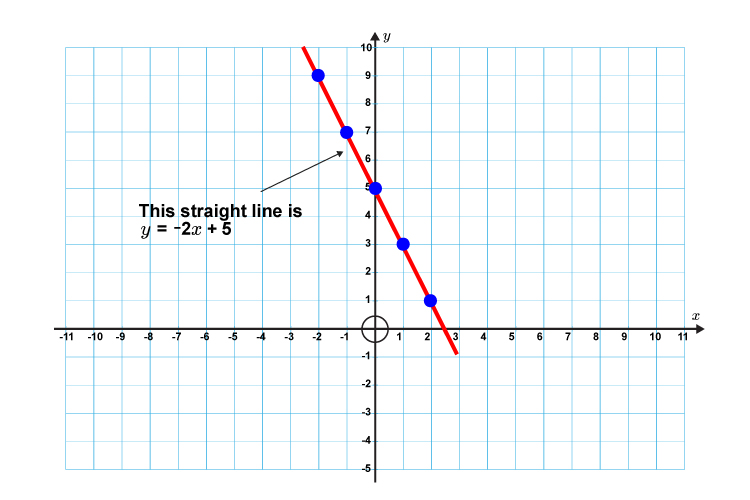# Formula for a straight line

The formula for a straight line is:

y=mx+c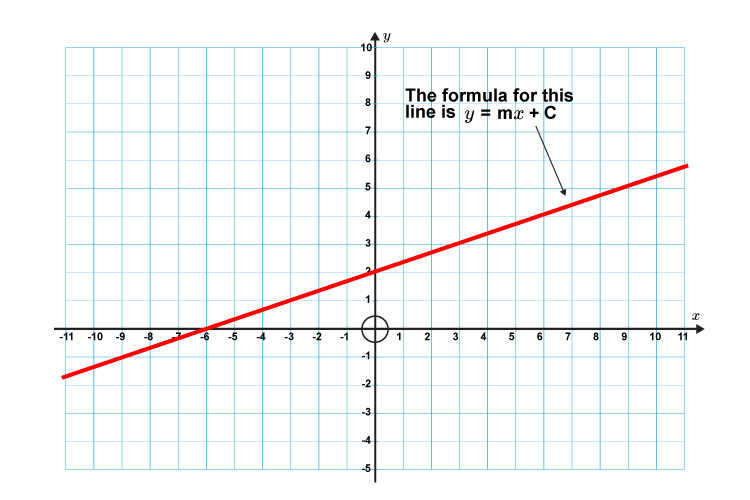Where m= Gradient

c= The point where the line crosses the y axis (y intercept)

## Further explanation

Equations of a straight line are in the form of:

y=mx+c

If you are given a formula of a straight line and asked to draw it on a graph you do so as follows:

Examples

1. Draw the following straight line on a graph

y=2x+3

First lets calculate some points on the graph.

If     x=-2               then   y=2times(-2)+3=-1

x=-1               then   y=2times(-1)+3=1

x=0                     then   y=2times0+3\ \ \ \ \ \ \ \ =3

x=1                     then   y=2times1+3\ \ \ \ \ \ \ \ =5

x=2                     then   y=2times2+3\ \ \ \ \ \ \ \ =7

Now draw a graph and plot the above points.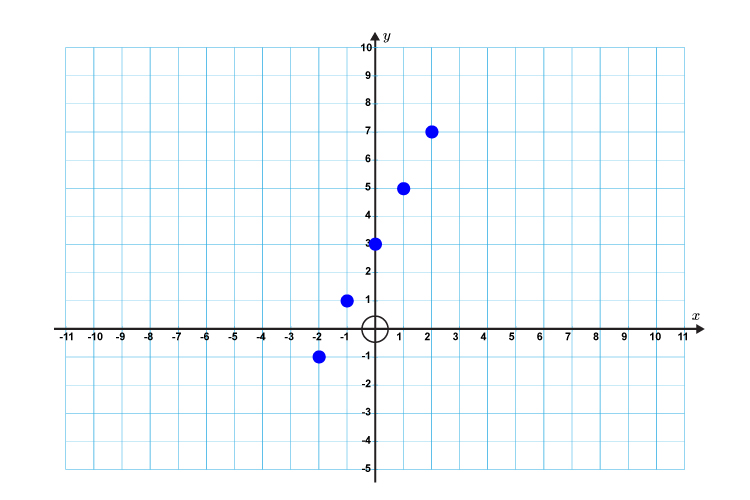Now draw a straight line through these points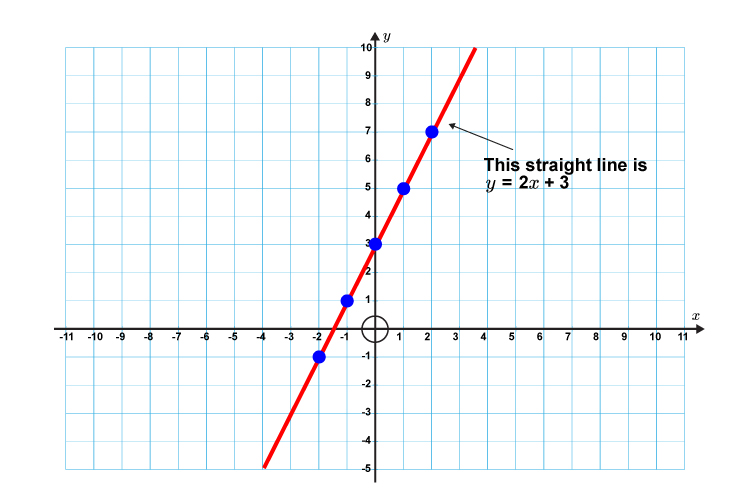2. Draw the following straight line on a graph

y=-2x+5

First lets calculate some points on the graph.

If     x=-2               then   y=-2times(-2)+5=9

x=-1               then   y=-2times(-1)+5=7

x=0                     then   y=-2times0+5\ \ \ \ \ \ \ \ =5

x=1                     then   y=-2times1+5\ \ \ \ \ \ \ \ =3

x=2                     then   y=-2times2+5\ \ \ \ \ \ \ \ =1

Now draw a graph and mark out the above points.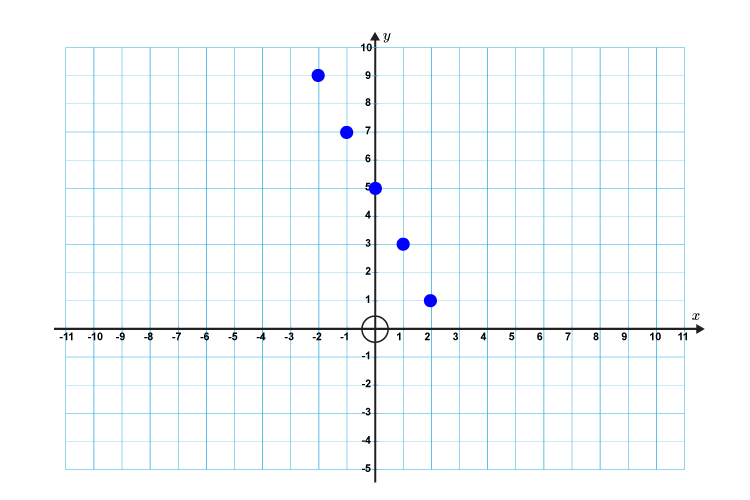Now draw a straight line through these points